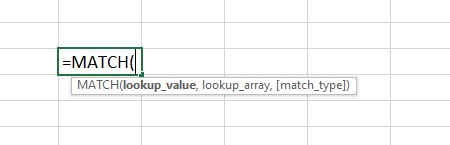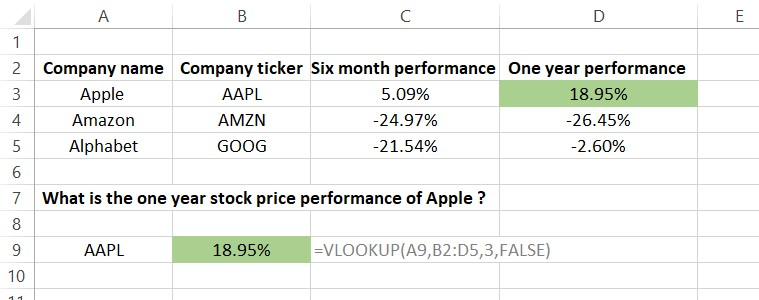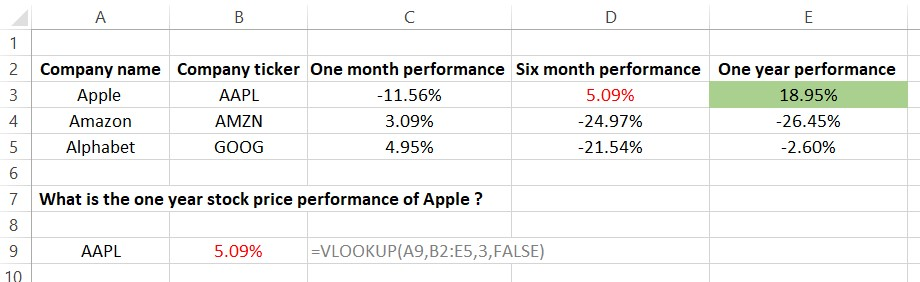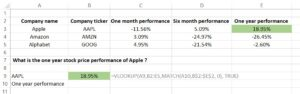## How to use the VLOOKUP and MATCH functions together

The VLOOKUP and MATCH functions are both used to search within Excel, and they can be used together to create a dynamic function that can overcome certain challenges.

The VLOOKUP function is used to search for information in a specified table. After inserting the row and column into the function, the formula returns the information in the overlapping cell.

The MATCH function plays a similar role in searching for information. However, its job is to return the number of the row or column where the input information is found.

The search criteria inserted into the VLOOKUP function is static, meaning that the function relies on the data source to remain unchanged in order to work correctly. The VLOOKUP fails to return any result or may return the incorrect result if the source data is altered in any way. This is one of the limitations of VLOOKUP.

To overcome this issue, the function can be used. The Match function used within the vlookup formula gives it a name of the column to search rather than just a fixed number of the column to search. This helps create a function that is more flexible. When VLOOKUP and MATCH are used together, the function becomes known as a dynamic function.

SyntaxThe VLOOKUP function appears in Excel as: =Vlookup(lookup_value, table_array, Col_index_number, [range_lookup], FALSE)

The simplified explanation of each of those is as follows:

• lookup_value: what you want to look up, Excel will search in the first column of the selected table.
• table_array: the table in which you wish to look up
• col_index_number: the number of the column to be searched, within the range that you selected.
• range_lookup: there are two options that can be selected in this section, FALSE or TRUE. This will tell Excel if it needs to look for the exact figure (FALSE) or an approximate figure (TRUE). False can also be written as 0 and true can also be written as 1.

VLOOKUP will return the value in the overlapping column and row cell, as specified in the formula.The MATCH function appears in Excel as: =MATCH(lookup_value, lookup_array, [match_type])

• lookup_value: what you want to look up, Excel will search in the row or column specified in the lookup_array
• lookup_array: the row or column in which you wish to look up
• match_type: three options to be selected in this section, depending on whether you want the returned number to be an exact match (0), less than (-1) or more than (+1) the lookup value.

The MATCH function will then return the number where the lookup value is within the lookup array.

Key Learning Points

• VLOOKUP and MATCH can both be used when searching for information in Excel.
• VLOOKUP returns information in a certain cell.
• The MATCH function returns the number of row or column.
• They both can be used together to form a dynamic function.
• Dynamic functions allow changes to be made to the raw data without impacting the results.

## The Limitations of VLOOKUP

The example below demonstrates how VLOOKUP can be used to retrieve data on a specific stock’s price performance. The VLOOKUP function returns the information in the relevant cell.However, in the example below we can see the situation in which our existing VLOOKUP fails.

After adding an additional column of “one month performance”, the VLOOKUP comes back with the incorrect information.Ideally, once a new column was added, the VLOOKUP should have updated to move its search criteria to the correct column, and this is exactly what the inclusion of the MATCH function helps to achieve.

## How to Use the MATCH Function within VLOOKUP

To prevent your formula from returning incorrect information in situations where data may be altered, the MATCH function can be integrated into VLOOKUP to make the function tolerate such changes.

As seen in the example below, by using the MATCH function within the VLOOKUP function, Excel now looks for which column the information is in (the MATCH function), and then returns the information in the correct cell (the VLOOKUP function)